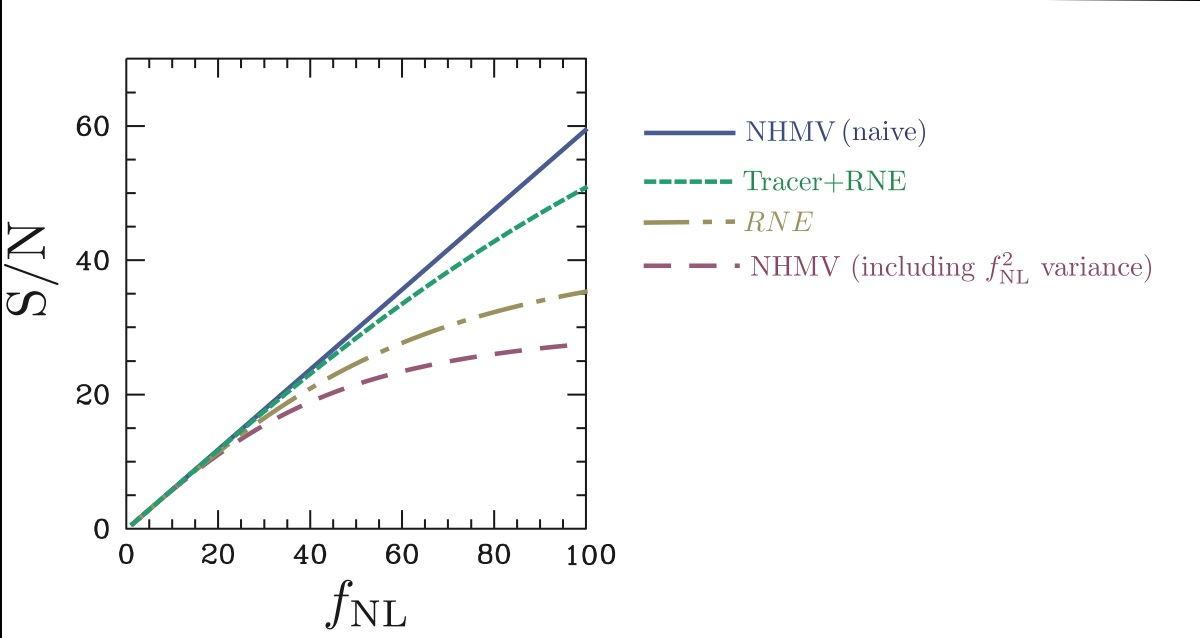# Estimators of primordial non-Gaussianity

Prior to the Planck satellite mission, analyses of WMAP satellite and large-scale structure data hinted at the presence of large local-type primordial non-Gaussianity with$f_{\rm NL}=30-100$. If this were the case [these hints were not confirmed by subsequent analyses of Planck satellite data], a large additional variance results in the null-hypothesis minimum variance estimator of$f_{\rm NL}$. We built on past work to reduce this variance using measurements of the 4-pt function, including for the first time a proper treatment of the radiation transfer function. The motivation was to prepare for the possibility that Planck would confirm hints of large local-type non-Gaussianity. We find that the late-time integrated Sachs-Wolfe (ISW) effect puts a fundamental limit on such techniques.

Results published in arXiv:1211.3417 (Phys. Rev. D 87, 063003).

Collaborators: Tristan Smith and Marc Kamionkowski.

Non-Gaussianity at the primordial level would be evidence for self-interaction in the field seeding the perturbations. One type of primordial non-Gaussianity is the so-called local model, in which$\Phi(\vec{x})=\phi_{G}(\vec{x})+f_{\rm NL}^{2}\left\{\phi_{G}^{2}(\vec{x})-\left \langle \phi_{G}^{2}(\vec{x})\right \rangle \right\}$

where$\Phi$ is the actual primordial potential perturbation,$\phi_{G}$ is an auxiliary Gaussian field, and${f}_{NL}$  is a number parameterizing the level of non-Gaussianity. This is more than a phenomenological possibility. Local-type non-Gaussianity should arise in the curvaton model, or if CDM axions make up a large fraction of the dark matter.
Estimators for local-type${f}_{NL}$ from the CMB rely first and foremost on the observed 3-pt correlation function of temperature and polarization anisotropies, and surprisingly become sub-optimal for high signal levels, with variance proportional to$f_{\rm NL}^{2}\left[\ln{\left(\frac{l_{\rm max}}{l_{\rm min}}\right)} \right]^{-2}$Signal to noise of estimators of primordial local-type non-Gaussianity, including the full radiation transfer function. The blue line corresponds to the naive variance of the null-hypothesis minimum variance estimator, while the purple line includes the additional variance which arises when non-Gaussianity is large. The green curve shows the signal-to-noise when some of the extra variance is removed by applying data from the 4-point function, while the aquamarine curve shows the result when a foreground tracer at redshift z~1-2 is used to remove contamination from the late-time Integrated Sachs-Wolfe (ISW) effect.

due to the much larger number of terms contributing to the variance than the signal in the relevant ensemble averages. This problem is particular to the local model, and Creminelli, Senatore, and Zaldarriaga suggested a clever way around the problem using the 4-pt function in astro-ph/0606001. Their technique was derived in the flat-sky, Sachs-Wolfe limit, completely neglecting the rich physics of the CMB transfer function.
We generalized their renormalized estimator using the appropriate full-sky expressions and including all the physics of the CMB transfer function. We also wrote the new estimator down in a way amenable to rapid computation from filtered CMB sky maps. We then assessed the new estimator, and find that the clever trick fails drastically in the real universe, due to the late-time ISW effect, which only allows removal of 50% of the piece of the variance that is dependent on${f}_{NL}$. We then showed that maps cleaned of the late-time ISW effect using a foreground tracer can more successfully remove the extra variance, as seen in the below figure:

In future work, we hope to generalize these techniques to polarization, and understand if the sub-optimal performance of the renormalized estimator(without use of foreground tracers) can be understood from first principles using the likelihood, and to make the connection with Bayesian treatments of the same estimation problem. We would also like to identify other areas of cosmology (weak gravitational lensing, estimation of number of relativistic species) where similar problems arise and similar methods could be fruitfully applied. As it turns out, Planck found very small local-type non-Gaussianity, in contrast with past results, so this line of inquiry has been tabled until nature or experiment calls for it to be further developed!